Request a Free Counselling Session from our Expert Mentor# NCERT Solution for Class 12 Math Chapter 4

NCERT Solutions for Class 12 Maths Chapter 4 “Determinants” is a crucial chapter for students who wish to pursue higher mathematics. In this chapter, students will learn about determinants, which are a mathematical tool used to solve linear equations, find the inverse of matrices, and solve a variety of mathematical problems.
The chapter begins by introducing the concept of determinants and its properties. Students will learn how to calculate determinants of matrices of different sizes and the properties of determinants, such as the multiplicative property and the triangular property.
NCERT Solutions for Class 12 Maths Chapter 4 also provide detailed explanations and a variety of solved examples on how to evaluate determinants using different methods such as expansion along rows or columns, minors and cofactors, and properties of determinants. Additionally, the solutions offer step-by-step guidance on how to approach different types of problems and help students to develop problem-solving skills.

## Answers of Mathematics NCERT solutions for class 12 Chapter 4 Determinants

Chapter 4

Determinants

Exercise 4.1

Question 1:

Evaluate the determinants in Exercises 1 and 2.

= 2(−1) − 4(−5) = − 2 + 20 = 18

Question 2:

Evaluate the determinants

(i)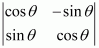(ii)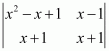(i)= (cos θ)(cos θ) − (−sin θ)(sin θ) = cos2 θ+ sin2 θ = 1

(ii)= (x2 − x + 1)(x + 1) − (x − 1)(x + 1)

x3 − x2 + x + x2 − x + 1 − (x2 − 1)

x3 + 1 − x2 + 1

x3 − x2 + 2

Question 3:

If then show that

The given matrix is

Question 4:

If then show that

The given matrix is.

It can be observed that in the first column, two entries are zero. Thus, we expand along the first column (C1) for easier calculation.

From equations (i) and (ii), we have:

Hence, the given result is proved.

Question 5:

Evaluate the determinants

(i) Let

It can be observed that in the second row, two entries are zero. Thus, we expand along the second row for easier calculation.

(ii) Let

By expanding along the first row, we have:

(iii) Let

By expanding along the first row, we have:

(iv) Let

By expanding along the first column, we have:

Question 6:

If , find

Let

By expanding along the first row, we have:

Question 7:

Find values of x, if

(i)

(ii)

(i)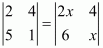(ii)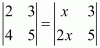Question 8:

If then x is equal to

(A) 6 (B) ±6 (C) −6 (D) 0

Hence, the correct answer is B.

Exercise 4.2

*Question 1:

Using the property of determinants and without expanding, prove that:

Question 2:

Using the property of determinants and without expanding, prove that:

Here, the two rows R1 and R3 are identical.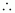Δ = 0.

Question 3:

Using the property of determinants and without expanding, prove that:

*Question 4:

Using the property of determinants and without expanding, prove that:

By applying C→ C3 + C2, we have:

Here, two columns C1 and Care proportional.Δ = 0.

Question 5:

Using the property of determinants and without expanding, prove that:

Applying R2 → R2 − R3, we have:

Applying R1 ↔R3 and R2 ↔R3, we have:

Applying R→ R1 − R3, we have:

Applying R1 ↔R2 and R2 ↔R3, we have:

From (1), (2), and (3), we have:

Hence, the given result is proved.

Question 6:

By using properties of determinants, show that:

We have,

Here, the two rows R1 and Rare identical.

∴Δ = 0.

Question 7:

By using properties of determinants, show that:

Applying R→ R2 + R1 and R→ R3 + R1, we have:

Question 8:

By using properties of determinants, show that:

(i)

(ii)

(i)

Applying R1 → R1 − Rand R2 → R2 − R3, we have:

Applying R1 → R1 + R2, we have:

Expanding along C1, we have:

Hence, the given result is proved.

(ii) Let .

Applying C1 → C1 − Cand C2 → C2 − C3, we have:

Applying C1 → C1 + C2, we have:

Expanding along C1, we have:

Hence, the given result is proved.

*Question 9:

By using properties of determinants, show that:

Applying R2 → R2 − Rand R3 → R3 − R1, we have:

Applying R3 → R3 + R2, we have:

Expanding along R3, we have:

Hence, the given result is proved.

Question 10:

By using properties of determinants, show that:

(i)

(ii)

(i)

Applying R1 → R1 + R+ R3, we have:

Applying C2 → C2 − C1, C3 → C3 − C1, we have:

Expanding along C3, we have:

Hence, the given result is proved.

(ii)

Applying R1 → R1 + R+ R3, we have:

Applying C2 → C2 − Cand C3 → C3 − C1, we have:

Expanding along C3, we have:

Hence, the given result is proved.

Question 11:

By using properties of determinants, show that:

(i)

(ii)

(i)

Applying R1 → R1 + R+ R3, we have:

Applying C2 → C2 − C1, C3 → C3 − C1, we have:

Expanding along C3, we have:

Hence, the given result is proved.

(ii)

Applying C1 → C1 + C+ C3, we have:

Applying R2 → R2 − Rand R3 → R3 − R1, we have:

Expanding along R3, we have:

Hence, the given result is proved.

Question 12:

By using properties of determinants, show that:

Applying R1 → R1 + R+ R3, we have:

Applying C2 → C2 − Cand C3 → C3 − C1, we have:

Expanding along R1, we have:

Hence, the given result is proved.

*Question 13:

By using properties of determinants, show that:

Applying R1 → R1 + bRand R2 → R2 − aR3, we have:

Expanding along R1, we have:

Question 14:

By using properties of determinants, show that:

Taking out common factors ab, and c from R1, R2, and Rrespectively, we have:

Applying R2 → R2 − Rand R3 → R3 − R1, we have:

Applying C1 → aC1, C→ bC2, and C3 → cC3, we have:

Expanding along R3, we have:

Hence, the given result is proved.

Question 15:

Let A be a square matrix of order 3 × 3, then  is equal to

A.     B.     C.   D.

A is a square matrix of order 3 × 3.

Hence, the correct answer is C.

Question 16:

Which of the following is correct?

A.  Determinant is a square matrix.

B.  Determinant is a number associated to a matrix.

C.  Determinant is a number associated to a square matrix.

D.  None of these

We know that to every square matrix,  of order n. We can associate a number called the determinant of square matrix A, where  element of A.

Thus, the determinant is a number associated to a square matrix.

Hence, the correct answer is C.

Exercise 4.3

Question 1:

Find area of the triangle with vertices at the point given in each of the following:

(i) (1, 0), (6, 0), (4, 3)

(ii) (2, 7), (1, 1), (10, 8)

(iii) (−2, −3), (3, 2), (−1, −8)

(i) The area of the triangle with vertices (1, 0), (6, 0), (4, 3) is given by the relation,

(ii) The area of the triangle with vertices (2, 7), (1, 1), (10, 8) is given by the relation,

(iii) The area of the triangle with vertices (−2, −3), (3, 2), (−1, −8)

is given by the relation,

Hence, the area of the triangle is square units.

*Question 2:

Show that points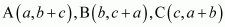are collinear

Area of ΔABC is given by the relation,

Thus, the area of the triangle formed by points A, B, and C is zero.

Hence, the points A, B, and C are collinear.

*Question 3:

Find values of k if area of triangle is 4 square units and vertices are

(i)    (k, 0), (4, 0), (0, 2) (ii)    (−2, 0), (0, 4), (0, k)

We know that the area of a triangle whose vertices are (x1y1), (x2y2), and

(x3y3) is the absolute value of the determinant (Δ), where

It is given that the area of triangle is 4 square units.

∴Δ = ± 4.

(i) The area of the triangle with vertices (k, 0), (4, 0), (0, 2) is given by the relation,

k + 4 = ± 4

When −k + 4 = − 4, k = 8.

When −k + 4 = 4, k = 0.

Hence, k = 0, 8.

(ii) The area of the triangle with vertices (−2, 0), (0, 4), (0, k) is given by the relation,

k − 4 = ± 4

When k − 4 = − 4, k = 0.

When k − 4 = 4, k = 8.

Hence, k = 0, 8.

Question 4:

(i) Find equation of line joining (1, 2) and (3, 6) using determinants

(ii) Find equation of line joining (3, 1) and (9, 3) using determinants

(i) Let P (xy) be any point on the line joining points A (1, 2) and B (3, 6). Then, the points A, B, and P are collinear. Therefore, the area of triangle ABP will be zero.

Hence, the equation of the line joining the given points is y = 2x.

(ii) Let P (xy) be any point on the line joining points A (3, 1) and

B (9, 3). Then, the points A, B, and P are collinear. Therefore, the area of triangle ABP will be zero.

Hence, the equation of the line joining the given points is x − 3y = 0.

Question 5:

If area of triangle is 35 square units with vertices (2, −6), (5, 4), and (k, 4). Then k is

A. 12  B. −2  C. −12, −2  D. 12, −2

The area of the triangle with vertices (2, −6), (5, 4), and (k, 4) is given by the relation,

It is given that the area of the triangle is ±35.

Therefore, we have:

When 5 − k = −7, k = 5 + 7 = 12.

When 5 − k = 7, k = 5 − 7 = −2.

Hence, k = 12, −2.

Exercise 4.4

Question 1:

Write Minors and Cofactors of the elements of following determinants:

(i)   (ii)

(i) The given determinant is .

Minor of element aij is Mij.

∴M11 = minor of element a11 = 3

M12 = minor of element a12 = 0

M21 = minor of element a21 = −4

M22 = minor of element a22 = 2

Cofactor of aij is Aij = (−1)i + j Mij.

∴A11 = (−1)1+1 M11 = (−1)2 (3) = 3

A12 = (−1)1+2 M12 = (−1)3 (0) = 0

A21 = (−1)2+1 M21 = (−1)3 (−4) = 4

A22 = (−1)2+2 M22 = (−1)4 (2) = 2

(ii) The given determinant is .

Minor of element aij is Mij.

∴M11 = minor of element a11 d

M12 = minor of element a12 b

M21 = minor of element a21 c

M22 = minor of element a22 a

Cofactor of aij is Aij = (−1)i + j Mij.

∴A11 = (−1)1+1 M11 = (−1)2 (d) = d

A12 = (−1)1+2 M12 = (−1)3 (b) = −b

A21 = (−1)2+1 M21 = (−1)3 (c) = −c

A22 = (−1)2+2 M22 = (−1)4 (a) = a

Question 2:

(i)   (ii)

(i) The given determinant is .

By the definition of minors and cofactors, we have:

M11 = minor of a11M12 = minor of a12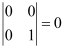M13 = minor of a13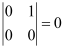M21 = minor of a21M22 = minor of a22M23 = minor of a23M31 = minor of a31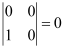M32 = minor of a32M33 = minor of a33A11 = cofactor of a11= (−1)1+1 M11 = 1

A12 = cofactor of a12 = (−1)1+2 M12 = 0

A13 = cofactor of a13 = (−1)1+3 M13 = 0

A21 = cofactor of a21 = (−1)2+1 M21 = 0

A22 = cofactor of a22 = (−1)2+2 M22 = 1

A23 = cofactor of a23 = (−1)2+3 M23 = 0

A31 = cofactor of a31 = (−1)3+1 M31 = 0

A32 = cofactor of a32 = (−1)3+2 M32 = 0

A33 = cofactor of a33 = (−1)3+3 M33 = 1

(ii) The given determinant is .

By definition of minors and cofactors, we have:

M11 = minor of a11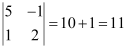M12 = minor of a12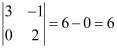M13 = minor of a13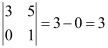M21 = minor of a21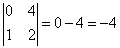M22 = minor of a22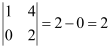M23 = minor of a23M31 = minor of a31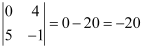M32 = minor of a32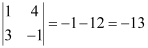M33 = minor of a33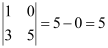A11 = cofactor of a11= (−1)1+1 M11 = 11

A12 = cofactor of a12 = (−1)1+2 M12 = −6

A13 = cofactor of a13 = (−1)1+3 M13 = 3

A21 = cofactor of a21 = (−1)2+1 M21 = 4

A22 = cofactor of a22 = (−1)2+2 M22 = 2

A23 = cofactor of a23 = (−1)2+3 M23 = −1

A31 = cofactor of a31 = (−1)3+1 M31 = −20

A32 = cofactor of a32 = (−1)3+2 M32 = 13

A33 = cofactor of a33 = (−1)3+3 M33 = 5

Question 3:

Using Cofactors of elements of second row, evaluate

The given determinant is

We have:

M21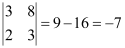∴A21 = cofactor of a21 = (−1)2+1 M21 = 7

M22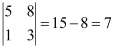∴A22 = cofactor of a22 = (−1)2+2 M22 = 7

M23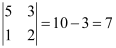∴A23 = cofactor of a23 = (−1)2+3 M23 = −7

We know that Δ is equal to the sum of the product of the elements of the second row with their corresponding cofactors.

∴Δ = a21A21 + a22A22 + a23A23 = 2(7) + 0(7) + 1(−7) = 14 − 7 = 7

Question 4:

Using Cofactors of elements of third column, evaluate

The given determinant is.

We have:

M13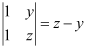M23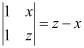M33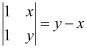∴A13 = cofactor of a13 = (−1)1+3 M13 = (z − y)

A23 = cofactor of a23 = (−1)2+3 M23 = − (z − x) = (x − z)

A33 = cofactor of a33 = (−1)3+3 M33 = (y − x)

We know that Δ is equal to the sum of the product of the elements of the second row with their corresponding cofactors.

Hence,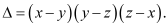Question 5:

If  and Aij is Cofactors of aij, then value of Δ is given by

We know that:

Δ = Sum of the product of the elements of a column (or a row) with their corresponding cofactors

∴Δ = a11A11 + a21A21 + a31A31

Hence, the value of Δ is given by the expression given in alternative D.

Exercise 4.5

Question 1:

Find adjoint of each of the matrices.

Question 2:

Find adjoint of each of the matrices.

Question 3:

Question 4:

Question 5:

Find the inverse of each of the matrices (if it exists).

Question 6:

Find the inverse of each of the matrices (if it exists).

Question 7:

Find the inverse of each of the matrices (if it exists).

*Question 8:

Find the inverse of each of the matrices (if it exists).

Question 9:

Find the inverse of each of the matrices (if it exists).

Question 10:

Find the inverse of each of the matrices (if it exists).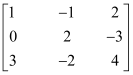.

*Question 11:

Find the inverse of each of the matrices (if it exists).

Question 12:

Let  and Verify that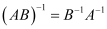From (1) and (2), we have:

(AB)−1 = B−1A−1

Hence, the given result is proved.

*Question 13:

If show that Hence find

Question 14:

For the matrix find the numbers a and b such that A2 + aA + bI O.

We have:

Comparing the corresponding elements of the two matrices, we have:

Hence, −4 and 1 are the required values of a and b respectively.

Question 15:

For the matrix show that A3 − 6A2 + 5A + 11 I = O. Hence, find A−1.

From equation (1), we have:

Question 16:

If  verify that A3 − 6A2 + 9A − 4I = O and hence find A−1

From equation (1), we have:

Question 17:

Let A be a nonsingular square matrix of order 3 × 3. Then |adj A|is equal to

A.   B.   C.   D.

We know that,

Hence, the correct answer is B.

Question 18:

If A is an invertible matrix of order 2, then det (A−1) is equal to

A. det (AB.    C. 1  D. 0

Since A is an invertible matrix,exists and

Hence, the correct answer is B.

Exercise 4.6

Question 1:

Examine the consistency of the system of equations.

+ 2= 2

2x + 3= 3

The given system of equations is:

+ 2= 2

2x + 3= 3

The given system of equations can be written in the form of AX = B, where

∴ A is non-singular.

Therefore, A−1 exists.

Hence, the given system of equations is consistent.

Question 2:

Examine the consistency of the system of equations.

2− y = 5

x + = 4

The given system of equations is:

2− y = 5

x + = 4

The given system of equations can be written in the form of AX = B, where

∴ A is non-singular.

Therefore, A−1 exists.

Hence, the given system of equations is consistent.

Question 3:

Examine the consistency of the system of equations.

x + 3y = 5

2x + 6y = 8

The given system of equations is:

x + 3y = 5

2x + 6y = 8

The given system of equations can be written in the form of AX = B, where

∴ A is a singular matrix.

Thus, the solution of the given system of equations does not exist. Hence, the system of equations is inconsistent.

Question 4:

Examine the consistency of the system of equations.

x + y z = 1

2x + 3y + 2z = 2

ax + ay + 2az = 4

The given system of equations is:

x + y z = 1

2x + 3y + 2z = 2

ax + ay + 2az = 4

This system of equations can be written in the form AX = B, where

∴ A is non-singular.

Therefore, A−1 exists.

Hence, the given system of equations is consistent.

Question 5:

Examine the consistency of the system of equations.

3x − y − 2z = 2

2y − z = −1

3x − 5y = 3

The given system of equations is:

3x − y − 2z = 2

2y − z = −1

3x − 5y = 3

This system of equations can be written in the form of AX = B, where

∴ A is a singular matrix.

Thus, the solution of the given system of equations does not exist. Hence, the system of equations is inconsistent.

Question 6:

Examine the consistency of the system of equations.

5x − y + 4z = 5

2x + 3y + 5z = 2

5x − 2y + 6z = −1

The given system of equations is:

5x − y + 4z = 5

2x + 3y + 5z = 2

5x − 2y + 6z = −1

This system of equations can be written in the form of AX = B, where

∴ A is non-singular.

Therefore, A−1 exists.

Hence, the given system of equations is consistent.

Question 7:

Solve system of linear equations, using matrix method.

The given system of equations can be written in the form of AX = B, where

Thus, A is non-singular. Therefore, its inverse exists.

Question 8:

Solve system of linear equations, using matrix method.

The given system of equations can be written in the form of AX = B, where

Thus, A is non-singular. Therefore, its inverse exists.

Question 9:

Solve system of linear equations, using matrix method.

The given system of equations can be written in the form of AX = B, where

Thus, A is non-singular. Therefore, its inverse exists.

Question 10:

Solve system of linear equations, using matrix method.

5x + 2y = 3

3x + 2y = 5

The given system of equations can be written in the form of AX = B, where

Thus, A is non-singular. Therefore, its inverse exists.

Question 11:

Solve system of linear equations, using matrix method.

The given system of equations can be written in the form of AX = B, where

Thus, A is non-singular. Therefore, its inverse exists.

Question 12:

Solve system of linear equations, using matrix method.

x − y + z = 4

2x + y − 3z = 0

x + y + z = 2

The given system of equations can be written in the form of AX = B, where

Thus, A is non-singular. Therefore, its inverse exists.

Question 13:

Solve system of linear equations, using matrix method.

2x + 3y + 3z = 5

x − 2y + z = −4

3x − y − 2z = 3

The given system of equations can be written in the form AX = B, where

Thus, A is non-singular. Therefore, its inverse exists.

Question 14:

Solve system of linear equations, using matrix method.

x − y + 2z = 7

3x + 4y − 5z = −5

2x − y + 3z = 12

The given system of equations can be written in the form of AX = B, where

Thus, A is non-singular. Therefore, its inverse exists.

*Question 15:

If find A−1. Using A−1 solve the system of equations

Now, the given system of equations can be written in the form of AX = B, where

*Question 16:

The cost of 4 kg onion, 3 kg wheat and 2 kg rice is Rs 60. The cost of 2 kg onion, 4 kg

wheat and 6 kg rice is Rs 90. The cost of 6 kg onion 2 kg wheat and 3 kg rice is Rs 70.

Find cost of each item per kg by matrix method.

Let the cost of onions, wheat, and rice per kg be Rs x, Rs y, and Rs z respectively.

Then, the given situation can be represented by a system of equations as:

This system of equations can be written in the form of AX = B, where

Now,

X = A−1 B

Hence, the cost of onions is Rs 5 per kg, the cost of wheat is Rs 8 per kg, and the cost of rice is Rs 8 per kg.

Miscellaneous Exercise

*Question 1:

Prove that the determinant is independent of θ.

Hence, Î” is independent of Î¸.

Question 2:

Without expanding the determinant, prove that

Hence, the given result is proved.

Question 3:

Expanding along C3, we have:

Question 4:

If ab and are real numbers, and

Show that either a + b + c = 0 or a = b = c.

Expanding along R1, we have:

Hence, if Δ = 0, then either a + b + c = 0 or a = b = c.

Question 5:

Solve the equations

Question 6:

Prove that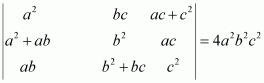Expanding along R3, we have:

Hence, the given result is proved.

Question 7:

If

We know that

Question 8:

Let  verify that

(i)

(ii)

(i)

We have,

(ii)

Question 9:

Evaluate

Question 10:

Evaluate

Expanding along C1, we have:

Question 11:

Using properties of determinants, prove that:

Expanding along R3, we have:

Hence, the given result is proved.

*Question 12:

Using properties of determinants, prove that:

Expanding along R3, we have:

Hence, the given result is proved.

Question 13:

Using properties of determinants, prove that:

Expanding along C1, we have:

Hence, the given result is proved.

Question 14:

Using properties of determinants, prove that:

Expanding along C1, we have:

Hence, the given result is proved.

Question 15:

Using properties of determinants, prove that:

Hence, the given result is proved.

*Question 16:

Solve the system of the following equations

Let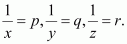Then the given system of equations is as follows:

This system can be written in the form of AX B, where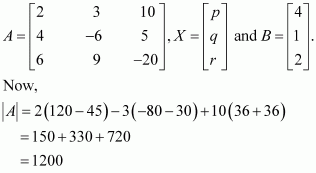A

Thus, A is non-singular. Therefore, its inverse exists.

Now,

A11 = 75, A12 = 110, A13 = 72

A21 = 150, A22 = −100, A23 = 0

A31 = 75, A32 = 30, A33 = − 24

Question 17:

If abc, are in A.P., then the determinant

A.   0  B.   1  C.   x  D.   2x

Here, all the elements of the first row (R1) are zero.

Hence, we have Δ = 0.

Question 18:

If xyz are nonzero real numbers, then the inverse of matrix is

A.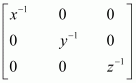B.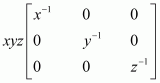C.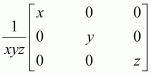D.

*Question 19:

Let , where 0 ≤ θ≤ 2π, then

A.  Det (A) = 0

B.  Det (A) ∈ (2, ∞)

C.  Det (A) ∈ (2, 4)

D.  Det (A)∈ [2, 4]

Now,

0 ≤ θ ≤ 2π

## Conclusion

NCERT Solutions for Class 12 Math Chapter 4 “Determinants” provide students with a comprehensive understanding of the concept of determinants and its properties. The chapter covers a variety of topics such as calculation of determinants, properties of determinants, and different methods to evaluate determinants.
The solutions offer clear explanations and numerous solved examples to help students grasp the concepts more effectively. The step-by-step guidance provided in the solutions also assists students in developing problem-solving skills.
Overall, NCERT Solutions for Class 12 Math Chapter 4 is an essential resource for students who want to excel in higher mathematics and develop their skills in solving complex mathematical problems. These solutions help students to build a strong foundation in the topic of determinants, which is a fundamental tool used in various fields of mathematics and science.

### Why opt for SWC?

One of the top IIT JEE coaching institutes is Swastik Classes. Shobhit Bhaiya and Alok Bhaiya, pioneering mentors of IIT JEE Coaching Classes, started Swastik Classes in Anand Vihar. Over the last 15 years, they have educated and sent over 2000+ students to IITs and 5000+ students to different famous universities such as BITS, NITs, DTU, and NSIT. When it comes to coaching programmes for IIT JEE, Swastik Classes is the top IIT JEE Coaching in Delhi, favoured by students from all over India.

Swastik Classes’ teachers have a solid academic background, having graduated from IIT with honours, and have extensive expertise in moulding students’ careers.

The study process in Swastik courses is separated between pre-class and post-class work, which is one of the most significant aspects. They are precisely created to improve the student’s mental ability and comprehension.

## Frequently Asked Questions on NCERT Solutions for Class 12 Maths Chapter 4

### How many problems are there in NCERT Solutions for Class 12 Maths Chapter 4?

Exercise 4.1 of NCERT Solutions has 8 questions, Exercise 4.2 has 16 questions, Exercise 4.3 and 4.4 has 5 questions, Exercise 4.5 has 18 questions, Exercise 4.6 has 16 questions and miscellaneous exercise has 19 questions. Long answers, short answers and MCQs are present in each exercise covering the topics which are important from the exam point of view. The solutions for the exercise wise problems are designed by a set of experts at BYJU’S having vast conceptual knowledge.

### What are the uses of determinants according to NCERT Solutions for Class 12 Maths Chapter 4?

Determinants is one of the main concepts having a number of applications in algebra. This concept is useful to solve a set of linear equations. Using determinants, students will be able to understand the change in area, volume, variables in terms of integrals. It can also be used to calculate the values of square matrices.

### What kind of problems can I expect in the board exams from NCERT Solutions for Class 12 Maths Chapter 4?

The problems based on solving the given set of linear equations using determinants can be expected from this chapter. Proofs that can be derived using the theory of determinants and prove that sums are also important from the exam perspective. In certain problems, you should determine the values of unknown variables using determinants and their resultant values.

### How many exercise determinants are there in class 12?

There are 6 Exercises in class 12 math determinants.

### What do you mean by determinants in mathematics?

The determinant is a scalar variable in mathematics that is a function of the elements of a square matrix. It allows you to characterize some of the matrix’s attributes as well as the linear map that the matrix represents.

### How do you find the minor of a matrix?

The determinant of each smaller matrix, produced by eliminating the appropriate rows and columns of each element in the matrix, is used to compute the minor of a matrix. We can create several minors of those matrices since there are many rows and columns with many elements in huge matrices.

### What is the determinant formula?

The determinant is: |A| = a (ei − fh) − b (di − fg) + c (dh − eg). The determinant of A equals ‘a times e x i minus f x h minus b times d x i minus f x g plus c times d x h minus e x g’.

### What if the determinant is 0?

If the determinant is zero, the matrix is not invertible and hence does not have a solution since one of the rows in the matrix may be deleted by substituting another row in the matrix.

### How do you solve a determinant equation?

A matrix is often used to represent the coefficients in a system of linear equations, and the determinant can be used to solve those equations.